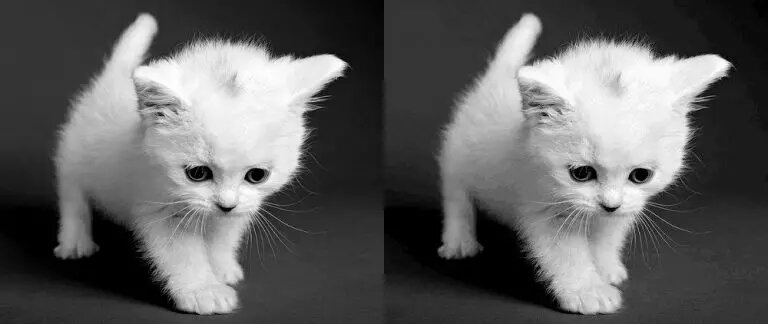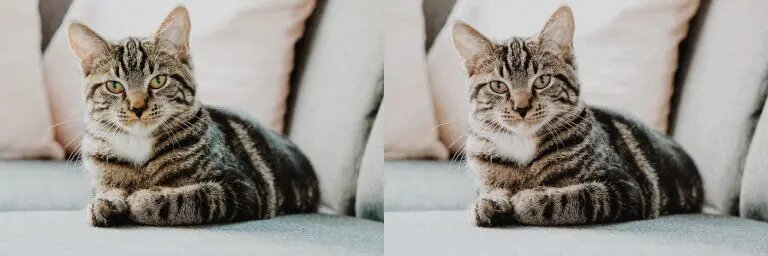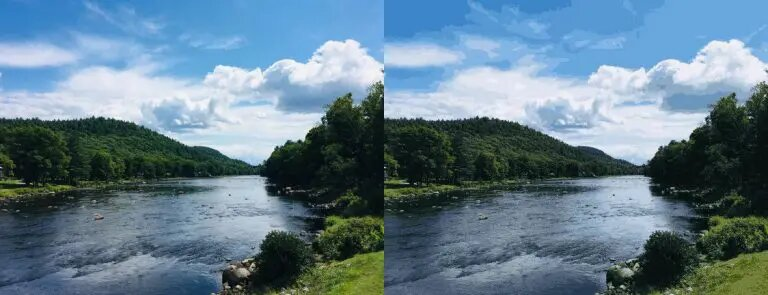# Color quantization using K-means clustering in ML.NET

When I was looking for K-means use cases, I found out about Color quantization, a very interesting . I implemented it in Python and was wondering whether it would be as easy to implement in ML.NET.

All the code is available in this GitHub repository.

## What is color quantization

Color quantization is the usage of quantization, a lossy compression technique, in color spaces in order to reduce the number of unique colors in an image.

A colorful image reduced to 4 colors using spatial color quantization.

## The use of K-means

K-means is an unsupervised clustering technique used to group N points into K clusters. In the past, it was computationally expensive to use it for quantization, until these recent years, as demonstrated by M. Emre Celebi.

1. Firstly, we load the RGB image and normalize the values (divide them by 255).
2. Secondly, we execute K-means on a sample of the data (with K equal to the number of colors we want the new image to have).
3. Lastly, we reconstruct the old image using the centroids of K-means. If we execute K-means with K=32, the new image will only use 32 colors.

## Implementation in ML.NET

First, we'll create a couple of classes to hold our data. It is useful to hold on to the width and height of the image so we can reconstruct the image later.

``````public class PixelEntry
{

// Normalized RGB values, e.g. [0.02323, 0.23013, 0.359305]
[VectorType(3)]
public float[] Features { get; set; }

}

public class ImageEntry
{

public PixelEntry[] Data { get; set; }
public int Width { get; set; }
public int Height { get; set; }

}

public class Prediction
{

public uint PredictedLabel { get; set; }

}
``````

Then we'll add a method to load the image. For this, we'll be using the SixLabors.ImageSharp package.

``````private static ImageEntry LoadImage(FileInfo file)
{
{
var pixels = new PixelEntry[img.Width * img.Height];

int i = 0;
foreach (var pixel in img.GetPixelSpan())
{
pixels[i++] = new PixelEntry
{
Features = new[]
{
(float)pixel.R / 255.0f,
(float)pixel.G / 255.0f,
(float)pixel.B / 255.0f
}
};
}

return new ImageEntry
{
Data = pixels,
Width = img.Width,
Height = img.Height
};
}
}
``````

The method takes as input the image (file) to load and returns the loaded image, with normalized data, as an `ImageEntry` instance.

### Training K-means

This step is made very easy by ML.NET. The input is the data and the number of clusters, and the output is a trained model.

``````private static ClusteringPredictionTransformer<KMeansModelParameters> Train(MLContext mlContext, IDataView data, int numberOfClusters)
{
var pipeline = mlContext.Clustering.Trainers.KMeans(numberOfClusters: numberOfClusters);

Console.WriteLine("Training model...");
var sw = Stopwatch.StartNew();
var model = pipeline.Fit(data);
Console.WriteLine("Model trained in {0} ms.", sw.Elapsed.Milliseconds);

return model;
}
``````

We also calculate the time taken when training to see how this technique performs.

### Reconstructing our image

Once the model is trained on the sample data, we can reconstruct our previous image using the new unique colors.

``````private static Image<Rgba32> ReconstructImage(Prediction[] labels, VBuffer<float>[] centroidsBuffer, int width, int height)
{
var img = new Image<Rgba32>(null, width, height);
int i = 0;
for (var h = 0; h < height; h++)
{
for (var w = 0; w < width; w++)
{
var label = labels[i].PredictedLabel;
var centroid = centroidsBuffer[label - 1].DenseValues().ToArray();
img[w, h] = new Rgba32(centroid, centroid, centroid);
i++;
}
}

return img;
}

private static void SaveImage(Image<Rgba32> image, FileInfo file)
{
using (var fs = new FileStream(file.FullName, FileMode.Create, FileAccess.Write))
{
image.SaveAsJpeg(fs);
}
}
``````

The predictions are basically which cluster this data belongs to. We use this information to select the centroid of that cluster and use it as a color.

The `Rgba32` constructor can handle values between 0 and 1 for RGB, but you can also multiply them by 255 if you want to.

### Putting everything together

``````private static void Main(string[] args)
{
var inputFile = new FileInfo("test3.jpg");
var outputFile = new FileInfo("result3.jpg");

var mlContext = new MLContext();

var model = Train(mlContext, trainingData, numberOfClusters: 32);

VBuffer<float>[] centroidsBuffer = default;
model.Model.GetClusterCentroids(ref centroidsBuffer, out int k);

var labels = mlContext.Data
.CreateEnumerable<Prediction>(model.Transform(fullData), reuseRowObject: false)
.ToArray();

Console.WriteLine("Reconstructing image...");
using var reconstructedImg = ReconstructImage(labels, centroidsBuffer, img.Width, img.Height);
SaveImage(reconstructedImg, outputFile);

Console.WriteLine("Original size: {0:F2} KB.", inputFile.Length / 1024.0);
Console.WriteLine("Result size: {0:F2} KB.", outputFile.Length / 1024.0);
}

private static T[] SelectRandom<T>(T[] array, int count)
{
var result = new T[count];
var rnd = new Random();
var chosen = new HashSet<int>();

for (var i = 0; i < count; i++)
{
int r;
while (chosen.Contains((r = rnd.Next(0, array.Length))))
{
continue;
}

result[i] = array[r];
}

return result;
}
``````

## ResultsOriginal (Left, 62 KB) vs Compressed (Right, 25 KB) using K=32 in ~200msOriginal (Left, 127 KB) vs Compressed (Right, 89 KB) using K=64 in ~500ms

Yes, I like cats.Original (Left, 58 KB) vs Compressed (Right, 49 KB) using K=32 in ~300ms

In this last image, we don't gain much space (~9KB) and the quality of the result image is obviously pixelized, increasing K to 64 would definitely help.

## Conclusion

Color quantization is an interesting technique that can be used to compress images, especially thumbnails to save a good amount of disk space in small amount of time (milliseconds).

We can use K-means for this technique, which performs well and fast and is fairly easy to implement. ML.NET ended up a lot easier to use than I anticipated and I can't wait to try it on other tasks!

Zanid Haytam is an enthusiastic programmer that enjoys coding, reading code, hunting bugs and writing blog posts.